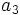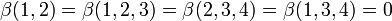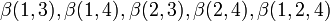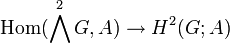# Second cohomology group for trivial group action of V4 on Z4

(diff) ← Older revision | Latest revision (diff) | Newer revision → (diff)
Jump to: navigation, search
This article gives information about the second cohomology group for trivial group action (i.e., the second cohomology group with trivial action) of the group Klein four-group on cyclic group:Z4. The elements of this classify the group extensions with cyclic group:Z4 in the center and Klein four-group the corresponding quotient group. Specifically, these are precisely the central extensions with the given base group and acting group.
The value of this cohomology group is elementary abelian group:E8.
Get more specific information about Klein four-group |Get more specific information about cyclic group:Z4|View other constructions whose value is elementary abelian group:E8

## Description of the group

We consider here the second cohomology group for trivial group action of the Klein four-group on cyclic group:Z4, i.e.,$\! H^2(G;A)$

where$G \cong V_4 \cong \mathbb{Z}_2 \times \mathbb{Z}_2$ and$A \cong \mathbb{Z}_4$.

The cohomology group is isomorphic to elementary abelian group:E8. As a vector space over field:F2, it is a three-dimensional vector space.

## Computation in terms of group cohomology

The cohomology group can be computed as an abstract group using the group cohomology of Klein four-group, which in turn can be computed using the Kunneth formula for group cohomology combined with the group cohomology of cyclic group:Z2.

We explain here the part of the computation based on the group cohomology of Klein four-group. As per that page, we have:$H^2(G;A) = (\operatorname{Ann}_A(2)) \oplus (A/2A)^2$

Here,$A/2A$ is the quotient of$A$ by$2A = \{ 2x \mid x \in A \}$ and$\operatorname{Ann}_A(2) = \{ x \in A \mid 2x = 0 \}$.

In our case,$A = \mathbb{Z}/2\mathbb{Z}$, so we get that both$A/2A$ and$\operatorname{Ann}_A(2)$ are both isomorphic to$\mathbb{Z}/2\mathbb{Z}$. Plugging in, we get:$H^2(G;A) = \mathbb{Z}/2\mathbb{Z} \oplus (\mathbb{Z}/2\mathbb{Z})^2 = (\mathbb{Z}/2\mathbb{Z})^3$

which is the elementary abelian group of order eight.

## Elements

### Summary

FACTS TO CHECK AGAINST (second cohomology group for trivial group action):
Background reading on relationship with extension groups: Group extension problem
Arithmetic functions of extension group:
order (thus all extension groups have the same order): order of extension group is product of order of normal subgroup and quotient group
nilpotency class: nilpotency class of extension group is between nilpotency class of quotient group and one more for central extension
derived length: derived length of extension group is bounded by sum of derived length of normal subgroup and quotient group
minimum size of generating set: minimum size of generating set of extension group is bounded by sum of minimum size of generating set of normal subgroup and quotient group|minimum size of generating set of quotient group is at most minimum size of generating set of group
WHAT'S THE TABLE BELOW?: Recall that there is a correspondence:
Elements of the group$H^2(G;A)$ for the trivial group action$\leftrightarrow$ congruence classes of central extensions with the specified subgroup$A$ and quotient group$G$.
This descends to a correspondence:
Orbits for the group action of$\operatorname{Aut}(G) \times \operatorname{Aut}(A)$ on$H^2(G;A)$$\leftrightarrow$ pseudo-congruence classes of central extensions.
The table below breaks down the second cohomology group as a union of these orbits, with (as a general rule) each row describing one orbit, i.e., one "cohomology class type", aka one "pseudo-congruence class" of central extensions. The number of rows is the number of pseudo-congruence classes of central extensions.

We list here the elements, grouped by similarity under the action of the automorphism groups on both sides.

Cohomology class type Number of cohomology classes Corresponding group extension Second part of GAP ID (order is 16) Stem extension? Base characteristic in whole group? Nilpotency class of extension group Derived length of extension group Subgroup information for base normal subgroup
trivial 1 direct product of Z4 and V4 10 No No 1 1
symmetric and nontrivial 3 direct product of Z8 and Z2 5 No Yes 1 1
non-symmetric 3 M16 6 No Yes 2 2 center of M16
non-symmetric 1 central product of D8 and Z4 13 No Yes 2 2 center of central product of D8 and Z4
Total (4 rows) 8 (equals order of the cohomology group) -- -- -- -- -- -- --

### Explicit description and relation with power-commutator presentation

Consider an extension group$E$ with central subgroup isomorphic to$A$ (cyclic group:Z4) and quotient group$G$ isomorphic to Klein four-group. Denote by$\overline{a_1}, \overline{a_2}$ elements of$G$ that form a basis for$G$ (i.e., they are unequal non-identity elements). Denote by$a_3$ a generator for$A$ and by$a_4$ the element$a_3^2$.

Let$a_1,a_2$ be elements of$E$ that map to$\overline{a_1}, \overline{a_2}$ respectively.

Then,$a_1,a_2,a_3,a_4$ generate$E$. Moreover, we can construct a power-commutator presentation for$E$ using these generators. Specifically, we know that$[a_1,a_3] = [a_1,a_4] = e, a_3^2 = a_4, a_4^2 = e$ and$[a_1,a_2],a_1^2, a_2^2$ are all in the set$\{ e,a_3,a_3a_4,a_4 \}$. Moreover, we also have that$[a_1,a_2]^2 = [a_1^2,a_2]$ and this tells us that in fact$[a_1,a_2] \in \{ e, a_4 \}$. The upshot is that we know that$\beta(1,2) = \beta(1,2,3) = \beta(2,3,4) = \beta(1,3,4) = 0$ and we need to specify the values of$\beta(1,3), \beta(1,4), \beta(2,3), \beta(2,4), \beta(1,2,4)$. This is a total of five numbers.

Some further whittling down is necessary. Note that by replacing$a_1$ by$a_1a_3$ if necessary, we can make sure that$\beta(1,4) = 0$. Similarly, by replacing$a_2$ by$a_2a_3$ is necessary, we can make sure that$\beta(2,4) = 0$. Thus, we have three values$\beta(1,3), \beta(2,3), \beta(1,2,4)$ that need to be specified. The mapping that sends a cohomology class to this triple of values, each viewed as an element of cyclic group:Z2, is an isomorphism.

The generic presentation we have is:$\langle a_1,a_2,a_3,a_4 \mid a_1^2 = a_3^{\beta(1,3)}, a_2^2 = a_3^{\beta(1,3)}, a_3^2 = a_4, a_4^2 = e, [a_1,a_2] = a_4^{\beta(1,2,4)}, [a_1,a_3] = [a_2,a_3] = [a_1,a_4] = [a_2,a_4] = [a_3,a_4] = e \rangle$

Based on the choices of$\beta(1,3), \beta(2,3), \beta(1,2,4)$, we get an actual presentation and an actual extension. The table below lists the possibilities:$\beta(1,3)$$\beta(2,3)$$\beta(1,2,4)$ Corresponding extension group Second part of GAP ID (order is 16) Nilpotency class
0 0 0 direct product of Z4 and V4 10 1
1 0 0 direct product of Z8 and Z2 5 1
0 1 0 direct product of Z8 and Z2 5 1
1 1 0 direct product of Z8 and Z2 5 1
0 0 1 central product of D8 and Z4 13 2
1 0 1 M16 6 2
0 1 1 M16 6 2
1 1 1 M16 6 2

## Group actions

### Action of automorphism group of acting group

By pre-composition, the automorphism group of the Klein four-group acts on the second cohomology group. Under this action, there are four orbits, corresponding to the four group extensions given above. Specifically, the 3 cohomology classes that give direct product of Z8 and Z2 are in one orbit, while the 3 cohomology classes that give M16 are in another orbit.

### Action of automorphism group of base group

The automorphism group of the base group has no effect on the cohomology classes. This is because this automorphism, the inverse map, pulls back trivially to the acting group, which has exponent two.

### Description of group actions in terms of explicit descriptions of elements

The automorphism group of the Klein four-group can be thought of as$2 \times 2$ matrices, and these naturally act on a two-dimensional vector space with basis$\beta(1,3), \beta(2,3)$. This is the desired action.$\beta(1,2,4)$ is fixed by the automorphism group.

## Subgroups of interest

Subgroup Value Corresponding group extensions for subgroup GAP IDs Group extension groupings for each coset GAP IDs
IIP subgroup of second cohomology group for trivial group action cyclic group:Z2 direct product of Z4 and V4 (1 copy) and central product of D8 and Z4 (1 copy) 10, 13 (direct product of Z4 and V4, central product of D8 and Z4) (1 copy) and (direct product of Z8 and Z2, M16) (3 copies) (10,13) (1 copy) and (5,6) (3 copies)
cyclicity-preserving subgroup of second cohomology group for trivial group action cyclic group:Z2 direct product of Z4 and V4 (1 copy), central product of D8 and Z4 (1 copy) 10, 13 (direct product of Z4 and V4, central product of D8 and Z4) (1 copy) and (direct product of Z8 and Z2, M16) (3 copies) (10,13) (1 copy) and (5,6) (3 copies)
subgroup generated by images of symmetric 2-cocycles (corresponds to abelian group extensions) Klein four-group direct product of Z4 and V4 (1 copy) and direct product of Z8 and Z2 (3 copies) 10, 5 (direct product of Z4 and V4, direct product of Z8 and Z2) and (central product of D8 and Z4, M16) (10,5) (1 copy) and (13,6) (1 copy)

## Direct sum decomposition

For background information, see formula for second cohomology group for trivial group action of abelian group in terms of Schur multiplier and abelianization

### General background

We know from the general theory that there is a natural short exact sequence:$0 \to \operatorname{Ext}^1_{\mathbb{Z}}(G,A) \to H^2(G;A) \stackrel{\operatorname{Skew}}{\to} \operatorname{Hom}(\bigwedge^2G,A) \to 0$

where the image of$\operatorname{Ext}^1$ is$H^2_{sym}(G;A)$, i.e., the group of cohomology classes represented by symmetric 2-cocycles (and corresponding to the abelian group extensions). We also know, again from the general theory, that the short exact sequence above splits, i.e.,$H^2_{sym}(G;A)$ has a complement inside$H^2$. However, there need not in general be a natural or even an automorphism-invariant choice of splitting.

### In this case

See also second cohomology group for trivial group action is internal direct sum of symmetric and cyclicity-preserving 2-cocycle subgroups if acting group is elementary abelian 2-group and every element of order two in the base group is a square

In terms of the general background, one way of putting this is that the skew map:$H^2(G;A) \stackrel{\operatorname{Skew}}{\to} \operatorname{Hom}(\bigwedge^2G,A)$

has a section (i.e., a reverse map):$\operatorname{Hom}(\bigwedge^2G,A) \to H^2(G;A)$

whose image is$H^2_{CP}(G;A)$ of cohomology classes represented by cyclicity-preserving 2-cocycles (see cyclicity-preserving subgroup of second cohomology group for trivial group action). Thus, the natural short exact sequence splits, and we get an internal direct sum decomposition:$H^2(G;A) = H^2_{sym}(G;A) + H^2_{CP}(G;A)$

A pictorial description of this is as follows. Here, each column is a coset of$H^2_{CP}(G,A)$ and each row is a coset of$H^2_{sym}(G,A)$. The top left entry is the identity element, hence the top row corresponds to abelian group extensions and the left column corresponds to cyclicity-preserving 2-cocycles.

The automorphism action acts as symmetric group:S3 on the three columns other than the left column, but preserves rows.

## Cocycles and coboundaries

### Size information

Group Order of group Isomorphism class of group Explanation
group of 1-cocycles for trivial group action$Z^1(G;A)$ 4 Klein four-group Same as$\operatorname{Hom}(G,A)$. see first cohomology group for trivial group action is naturally isomorphic to group of homomorphisms
group of all 1-cochains for trivial group action$C^1(G;A)$ 256 direct product of Z4 and Z4 and Z4 and Z4 all set maps from$G$ to$A$ with pointwise addition.
group of all 2-coboundaries for trivial group action$B^2(G;A)$ 64 direct product of Z4 and Z4 and V4 By the first isomorphism theorem and the definition of this group, it is isomorphic to the group (1-cochains)/(1-cocycles).
group of all 2-cocycles for trivial group action$Z^2(G;A)$ 512 PLACEHOLDER FOR INFORMATION TO BE FILLED IN: [SHOW MORE] PLACEHOLDER FOR INFORMATION TO BE FILLED IN: [SHOW MORE]
second cohomology group for trivial group action 8 elementary abelian group:E8 This is$Z^2/B^2$, so orders divide.

In particular, what this means is that for every cohomology class, there are 64 different choices of 2-cocycles that represent that cohomology class.

We give the corresponding information if we use normalized cocycles and coboundaries:

Group Order of group (equals 2 to the power of dimension) Isomorphism class of group Explanation
group of normalized 1-cocycles for trivial group action$Z^1_n(G;A)$ 4 Klein four-group Same as$\operatorname{Hom}(G,A)$. see first cohomology group for trivial group action is naturally isomorphic to group of homomorphisms
group of normalized 1-cochains for trivial group action$C^1_n(G;A)$ 64 direct product of Z4 and Z4 and Z4 all set maps from$G$ to$A$ with pointwise addition, that send the identity to the identity. There are thus 3 elements that can be mapped arbitrarily.
group of all normalized 2-coboundaries for trivial group action$B^2_n(G;A)$ 16 direct product of Z4 and V4 By the first isomorphism theorem and the definition of this group, it is isomorphic to the group (normalized 1-cochains)/(normalized 1-cocycles).
group of all normalized 2-cocycles for trivial group action$Z^2_n(G;A)$ 128 PLACEHOLDER FOR INFORMATION TO BE FILLED IN: [SHOW MORE]
second cohomology group for trivial group action 8 elementary abelian group:E8 This is$Z^2_n/B^2_n$.

In particular, what this means is that for every cohomology class, there are 16 different choices of normalized 2-cocycles that represent that cohomology class.

## Generalized Baer Lie rings

The examples here illustrate the cocycle halving generalization of Baer correspondence.

The direct sum decomposition (discussed in the preceding section):$H^2(G;A) = H^2_{sym}(G;A) + H^2_{CP}(G;A)$

gives rise to some examples of the cocycle halving generalization of Baer correspondence. For any group extension arising as an element of$H^2(G;A)$, the additive group of its Lie ring arises as the group extension corresponding to the projection onto$H^2_{sym}(G;A)$, and the Lie bracket coincides with the group commutator.

In the description below, the additive group of the Lie ring of a given group is the unique abelian group in the column corresponding to that group.

Thus, we have two correspondences emerging:

Group GAP ID Additive group of Lie ring GAP ID More about the correspondence
central product of D8 and Z4 (16,13) direct product of Z4 and V4 (16,10) generalized Baer correspondence between central product of D8 and Z4 and direct product of Z4 and V4
M16 (16,6) direct product of Z8 and Z2 (16,5) generalized Baer correspondence between M16 and direct product of Z8 and Z2